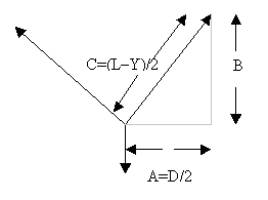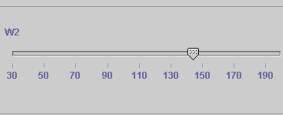search UMD   This siteUser’s Guide The model represents the Pulley-Weight mechanism where the position of the weights suspended at two different points can be determined by the weights of the box and the D distance as represented in the diagram below. From the geometry: A2 + B2 = C2 B= sqrt ((L –Y) 2 – D2) / 2   where (L – Y)/2 is the value of component C. The simulation model uses the equilibrium equation as follows: å FY = 2W2 * ( B/ (L – Y /2))- w1 = 0Moving the upper right corner of the pulley structure changes the distance D. On clicking at corners represented by points B, C, A, w1 and W2 the corresponding Free Body Diagrams are shown.The adjacent Figure represents the geometry of the mechanismUsing the above shown sliders following parameters can be changed: Weight w1 Weight W2